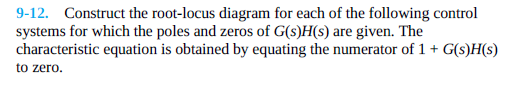# Question (1) Poles at j, –j, j2, –j2; zeros at –2, 2 (2) Poles at j, –j, j2, –j2; zeros at –1, 1 Please write down all calculations. Thanks. 9-12. Construct the root-locus diagram for each of the following control systems for which the poles and zeros of G(s)H(s) are given. The characteristic equation is obtained by equating the numerator of 1 + G(s)H(s) to zero.WWO8OB The Asker · Electrical Engineering(1) Poles at j, –j, j2, –j2; zeros at –2, 2
(2) Poles at j, –j, j2, –j2; zeros at –1, 1

Please write down all calculations. Thanks.

Transcribed Image Text: 9-12. Construct the root-locus diagram for each of the following control systems for which the poles and zeros of G(s)H(s) are given. The characteristic equation is obtained by equating the numerator of 1 + G(s)H(s) to zero.
More
Transcribed Image Text: 9-12. Construct the root-locus diagram for each of the following control systems for which the poles and zeros of G(s)H(s) are given. The characteristic equation is obtained by equating the numerator of 1 + G(s)H(s) to zero.